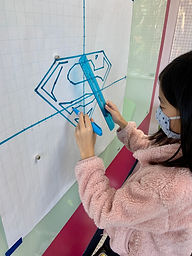## Mr. Rodney

### Target 1​

###### Lesson Type:

Review

Geometry

:

Coordinates

Use coordinates to find a location on a grid.

###### 1:

Identify the x- and y-axis on a coordinate plane.

###### 2:

Locate points in all four quadrants of the coordinate plane.

###### 3:

Recognize which quadrant a point is in, based on the properties of its coordinate pairs.

###### 4:

Understand that the first number in a coordinate pair indicates placement on the x-axis and the second indicates placement on the y-axis.

5th

###### Vocabulary:

Coordinates, X-/Y-Axis, Point, Ordered Pair

Activities:

• Reviewed and discussed vocabulary terms, defining them using words that are meaningful and accurate.
• Took turns plotting points on a coordinate plane, making sure to plot points in the exact order listed to create a shaps and connected them.
• Classified shapes being made by name based on the number of sides.
• Used critical thinking skills to determine what the overall image was before completing the activity.### Home Exploration

###### Guiding Questions:## Absent Students:

### Target 2

:

###### 1:

Understand that a function is a rule that gives one output to each input.

###### 2:

Understand that by studying the proportional relationship between inputs and outputs, a function (equation) can be derived.

###### 3:

Use a function equation to fill out a function table.

6th

###### Vocabulary:

Function, Input, Output, Function Rule

Activities:

• Students were introduced to the concept of "functions" by determining simple "function rules" that resulted from the "fuction machine.
• Students inserted drawings of basic shapes into one side of the function machine (input) and studied what happened when they came out, or the "output."
• The function rules were initially as simple as "added a happy face" or "colored the shapes blue."
• The exercised was then translated into numbers in which students each chose an input number, (x), and studied the output, f(x).
• Determined the function rule that applied to the numbers, such as "x-3."### Home Exploration

###### Guiding Questions:### Target 3

:

###### Vocabulary:

Activities:### Home Exploration